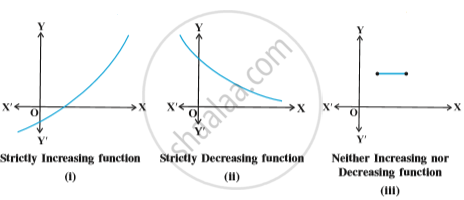# Increasing and Decreasing Functions

#### definition

Let I be an interval contained in the domain of a real valued function f. Then f is said to be
(i) increasing on I if x_1 < x_2 in I ⇒ f(x_1) < f(x_2) for all x_1, x_2 ∈ I.
(ii) decreasing on I, if x_1, x_2 in I ⇒ f(x_1) < f(x_2) for all x_1, x_2 ∈ I.
(iii) constant on I, if f(x) = c for all x ∈ I, where c is a constant.
(iv) decreasing on I if x_1 < x_2 in I ⇒ f(x_1) ≥ f(x_2) for all x_1, x_2 ∈ I.
(v) strictly decreasing on I if x_1 < x_2 in I ⇒ f(x_1) > f(x_2) for all  x_1, x_2 ∈ I.
For graphical representation of such functions see Fig.#### definition

Let x_0 be a point in the domain of definition of a real valued function  f. Then  f  is said to be increasing, decreasing at x_0 if  there exists an open interval I containing x_0 such that f is increasing, decreasing, respectively, in I.

#### theorem

Let  f  be continuous on [a, b] and differentiable on the open interval (a,b). Then
(a) f  is increasing in [a,b] if f′(x) > 0 for each x ∈  (a, b)
(b) f  is decreasing in [a,b] if f′(x) < 0 for each x ∈ (a, b)
(c) f  is a constant function in [a,b] if f′(x) = 0 for each x ∈ (a, b)

Proof:  (a) Let x_1, x_2 ∈ [a, b] be such that x_1 < x_2.
Then, by Mean Value Theorem (Theorem 8 in Chapter 5), there exists a point c between x_1 and x_2 such that
f(x_2) – f(x_1) = f′(c) (x_2 – x_1)
i.e. f(x_2) – f(x_1) > 0        (as f′(c) > 0 (given))

i.e. f(x_2) > f(x_1)

Thus, we have x_1< x_2 f(x_1) f(x_2) ,  for all x_1,x_2 [a,b]

Hence, f  is an increasing function in [a,b].
The proofs of part (b) and (c) are similar. It is left as an exercise to the reader.

If you would like to contribute notes or other learning material, please submit them using the button below.

#### Video Tutorials

We have provided more than 1 series of video tutorials for some topics to help you get a better understanding of the topic.

Series 1

Series 2

Series 3

### Shaalaa.com

Increasing Decreasing Functions Introduction Part 1 [00:37:26]
S
0%